When I first learned to program in SAS, I remember being confused about the difference between CLASS statements and BY statements. A novice SAS programmer recently asked when to use one instead of the other, so this article explains the difference between the CLASS statement and BY variables in SAS procedures.

The BY statement and the CLASS statement in SAS both enable you to specify one or more categorical variables whose levels define subgroups of the data. (For simplicity, we consider only a single categorical variable.) The primary difference is that the BY statement computes many analyses, each on a subset of the data, whereas the CLASS statement computes a single analysis of all the data. Specifically,

• The BY statement repeats an analysis on every subgroup. The subgroups are treated as independent samples. If a BY variable defines k groups, the output will contain k copies of every table and graph, one copy for the first group, one copy for the second group, and so on.
• The CLASS statement includes a categorical variable as part of an analysis. Often the CLASS variable is used to compare the groups, such as in a t test or an ANOVA analysis. In regression models, the CLASS statement enables you to estimate parameters for the levels of a categorical variable, thereby estimating the effect of each level on the response. Another use of a CLASS variable is to define categories for a classification task, such as a discriminant analysis.

To illustrate the differences between an analysis that uses a BY statement and one that uses a CLASS statement, let's create a subset (called Cars) of the Sashelp.Cars data. The levels of the Origin variable indicate whether a vehicle is manufactured in "Asia", "Europe", or the "USA". For efficiency reasons, most classical SAS procedures require that you sort the data when you use a BY statement. Therefore, a call to PROC SORT creates a sorted version of the data called CarsSorted, which will be used for the BY-group analyses.

```data Cars; set Sashelp.Cars; where cylinders in (4,6,8) and type ^= 'Hybrid'; run;   proc sort data=Cars out=CarsSorted; by Origin; run;```

### Descriptive statistics for grouped data

When you generate descriptive statistics for groups of data, the univariate statistics are identical whether you use a CLASS statement or a BY statement. What changes is the way that the statistics are displayed. When you use the CLASS statement, you get one table that contains all statistics or one graph that shows the distribution of each subgroup. However, when you use the BY statement you get multiple tables and graphs.

The following statements use the CLASS statement to produce descriptive statistics. PROC UNIVARIATE displays one (paneled) graph that shows a comparative histogram for the vehicles that are made in Asia, Europe, and USA. PROC MEANS displays one table that contains descriptive statistics:

```proc univariate data=Cars; class Origin; var Horsepower; histogram Horsepower / nrows=3; /* must use NROWS= to get panel */ ods select histogram; run;   proc means data=Cars N Mean Std; class Origin; var Horsepower Weight Mpg_Highway; run;```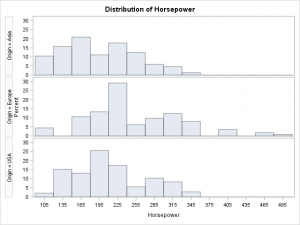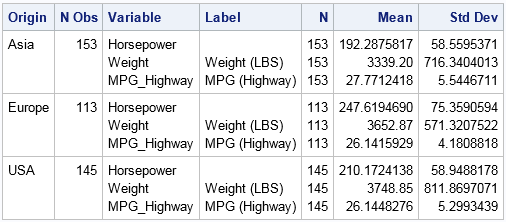In contrast, if you run a BY-group analysis on the levels of the Origin variable, you will see three times as many tables and graphs. Each analysis is preceded by a label that identifies each BY group. Notice that the BY-group analysis uses the sorted data.

```proc means data=CarsSorted N Mean Std; by Origin; var Horsepower Weight Mpg_Highway; run;```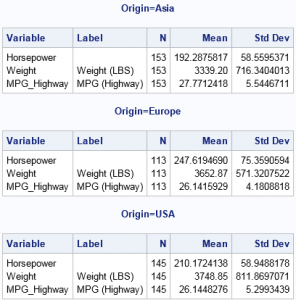Always remember that the output from a BY statement is equivalent to the output from running the procedure multiple times on subsets of the data. For example, the previous statistics could also be generated by calling PROC MEANS three times, each call with a different WHERE clause, as follows:

```proc means N Mean Std data=CarsSorted( where=(origin='Asia') ); var Horsepower Weight Mpg_Highway; run; proc means N Mean Std data=CarsSorted( where=(origin='Europe') ); var Horsepower Weight Mpg_Highway; run; proc means N Mean Std data=CarsSorted( where=(origin='USA') ); var Horsepower Weight Mpg_Highway; run;```

In fact, if you ever find yourself repeating an analysis many times (perhaps by using a macro loop), you should consider whether you can rewrite your program to be more efficient by using a BY statement.

### Comparing groups: Use the CLASS statement

As a general rule, you should use a CLASS statement when you want to compare or contrast groups. For example, the following call to PROC GLM performs an ANOVA analysis on the horsepower (response variable) for the three groups defined by the Origin variable. The procedure automatically creates a graph that displays three boxplots, one for each group. The procedure also computes parameter estimates for the levels of the CLASS variable (not shown).

```proc glm data=Cars; /* by default, create graph with side-by-side boxplots */ class Origin; model Horsepower = Origin / solution; run;```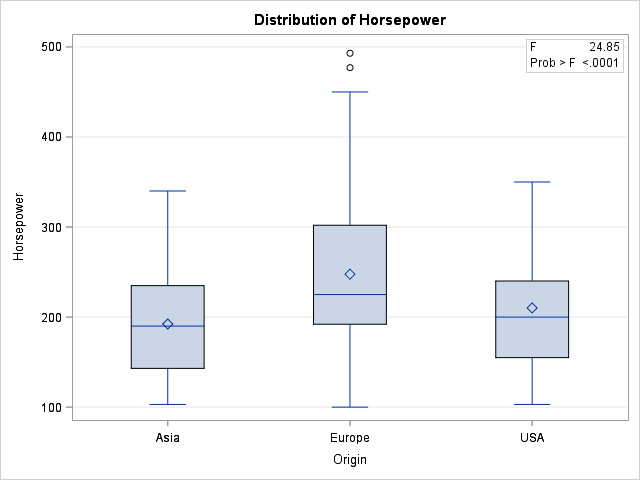You can specify multiple variables on the CLASS statement to include multiple categorical variables in a model. Any variables that are not listed on the CLASS statement are assumed to be continuous. Thus the following call to PROC GLM analyzes a model that has one continuous and one classification variable. The procedure automatically produces a graph that overlays the three regression curves on the data:

```ods graphics /antialias=on; title "CLASS Variable Regression: One Model with Multiple Parameters"; proc GLM data=Cars plots=FitPlot; class Origin; model Horsepower = Origin | Weight / solution; ods select ParameterEstimates ANCOVAPlot; quit;```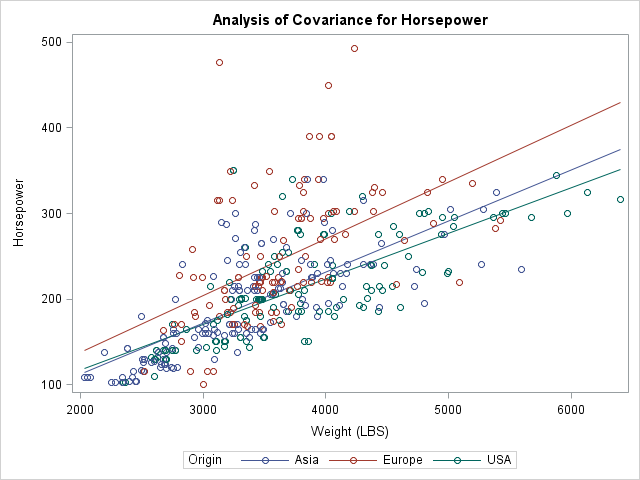In contrast, if you use a BY statement, the Origin variable cannot be part of the model but is used only to subset the data. If you use a BY statement, you obtain three different models of the form Horsepower = Weight. You get three parameter estimates tables and three graphs, each showing one regression line overlaid on a subset of the data.

### Predicted Values: CLASS VARIABLE versus BY Variable

When you use a BY statement and fit three models of the form Horsepower = Weight, the procedure fits a total of six parameters. Notice that when you use the CLASS statement and fit the model Horsepower = Origin | Weight, you also fit six free parameters. It turns out that these two methods produce the same predicted values. In fact, you can combine the parameter estimates (for the GLM parameterization) for the CLASS model to obtain the parameter estimates from the BY-variable analysis, as shown below. Each parameter estimate for the BY-variable models are obtained as the sum of two estimates for the CLASS-variable analysis: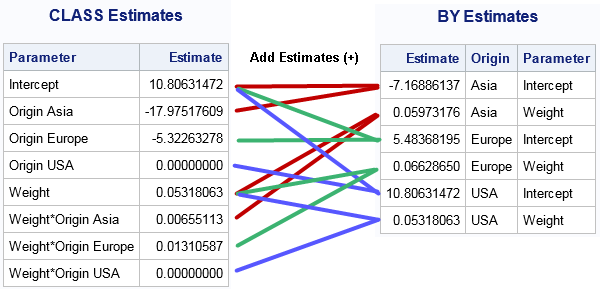For many regression models, the predicted values for the BY-variable analyses are the same as for a particular model that uses a CLASS variable. As shown above, you can even see how the parameters are related when you use a GLM or reference parameterization. However, the CLASS variable formulation can fit models (such as the equal-slope model Horsepower = Origin Weight) that are not available when you use a BY variable to fit three separate models. Furthermore, the CLASS statement provides parameter estimates so that you can see the effect of the groups on the response variable. It is more difficult to compare the models that are produced by using the BY statement.

### Other CLASS-like statements in SAS

Some SAS procedures use a different syntax to analyze groups. In particular, the SGPLOT procedure calls classification variables "group variables." If you want to overlay graphs for multiple groups, you can use the GROUP= option on many SGPLOT statements. (Some statements support the CATEGORY= option, which is similar.) For example, to replicate the two-variable regression analysis from PROC GLM, you can use the following statements in PROC SGPLOT:

```proc sgplot data=Cars; reg y=Horsepower x=Weight / group=Origin; /* Horsepower = Origin | Weight */ run;```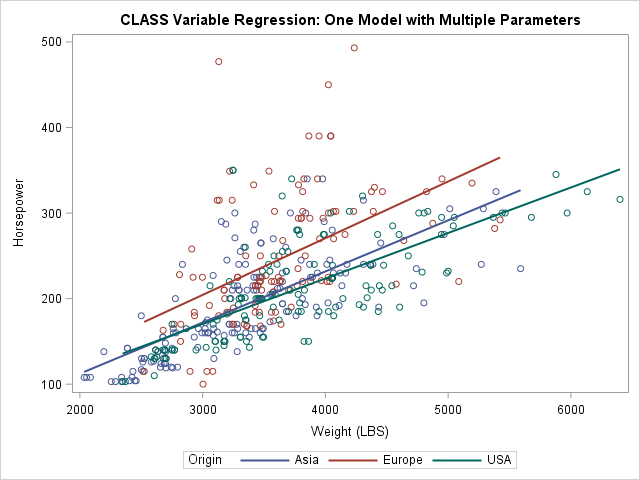### Summary

In summary, use the BY statement in SAS procedures when you want to repeat an analysis for every level of one or more categorical variables. The variables define the subsets but are not otherwise part of the analysis. In classical SAS procedures, the data must be sorted by the BY variables. A BY-group analysis can produce many tables and graphs, so you might want to suppress the ODS output and write the results to a SAS data set.

Use the CLASS statement when you want to include a categorical variable in a model. A CLASS statement often enables you to compare or contrast subgroups. For example, in regression models you can evaluate the relative effect of each level on the response variable.

In some cases, the BY statement and the CLASS statement produce identical statistics. However, the CLASS statement enables you to fit a wider variety of models.

ShareDistinguished Researcher in Computational Statistics

Rick Wicklin, PhD, is a distinguished researcher in computational statistics at SAS and is a principal developer of SAS/IML software. His areas of expertise include computational statistics, simulation, statistical graphics, and modern methods in statistical data analysis. Rick is author of the books Statistical Programming with SAS/IML Software and Simulating Data with SAS.

1.Chris Hemedinger on

Good primer on CLASS vs BY! I think that many new SAS programmers learn about the BY statement first because it applies more generally across SAS procedures. But in practice, CLASS is more elegant for the categorical analysis/reporting that we often need. And even if your data values are continuous or high-cardinality (which would be ill-suited for BY or CLASS), you can use a SAS format to create an instant classification variable. For example, if you have a datetime variable in a data set full of transactions, you can easily roll these up to yearly categories by applying the DTYEAR format. I published a tip for this specific to datetime values here.

•Thanks for the comment and tip. PROC FORMAT is definitely a powerful way to bin a continuous variable or collapse a high-cardinality variable into a smaller number of levels.

•Best explanation I have seen on the By and Class differences.

2.Very nice article, Rick! Great job!

3.Vishal Dhamal on

Very useful article. I am relatively new to SAS programming, so this will certainly help going forward.

•Welcome to the SAS family. Newcomers and veterans ask and answer questions at the SAS Support Communities. You might want to check them out!

4.Really very nice topic sir, clear to me the difference between class and by

5.Thanks Rick .can u post some other articles on like proc Freq

•I've posted many articles about PROC FREQ. Do an internet search for the terms that interest you, but restrict the search to my blog by using a search string such as

"proc freq" other-terms-here site:blogs.sas.com/content/iml

6.thank you for sharing the information, but could you please give a in detail difference between BY statement and Class statement.

thanks,
shekhar Anagandula

•I consider this article detailed, but if you have specific questions you can always ask them at the SAS Support Communities. I suggest the Community for Statistical Procedures.

7.Very informative and simple document. It is really very nice comparison between CLASS and BY variables with good examples. Thank you.